## Saturday, 9 August 2014

### chapter 1 Concept of Logarithms

Logarithms are a very convenient mathematical tool, used extensively to simplify calculations. In our experience, many students know how to use logarithms (logs for short), but do not know what they actually are. Therefore, this part should be read keeping in mind the aim that you need to understand the underlying idea behind logs before trying to put them to use.
Consider the following equation: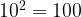${10^2} = 100$
This would be read as “Ten to the power two equals one hundred”. With logs, you can equivalently state this problem as: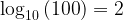${\log _{10}}\left( {100} \right) = 2$
which would be read as “The logarithm of hundred to the base ten is two.”
Consider some more examples: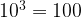${10^3} = 100$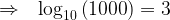$\Rightarrow \,\,\,\, {\log _{10}}\left( {1000} \right) = 3$ Read as: “The log of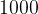$1000$ to base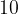$10$ is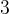$3$”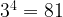${3^4} = 81$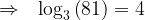$\Rightarrow \,\,\,\,{\log _3}\left( {81} \right) = 4$ Read as: “The log of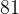$81$ to base$3$ is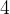$4$”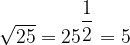$\sqrt {25} = {25^{\dfrac{1}{2}}} = 5$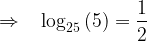$\Rightarrow \,\,\,\,\, {\log _{25}}\left( 5 \right) = \dfrac{1}{2}$ Read as: “The log of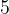$5$ to base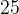$25$ is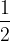$\dfrac{1}{2}$”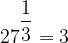${27^{\dfrac{1}{3}}} = 3$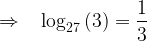$\Rightarrow \,\,\,\,\, {\log _{27}}\left( 3 \right) = \dfrac{1}{3}$ Read as: “The log of$5$ to base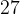$27$ is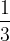$\dfrac{1}{3}$”
Thus, we see that the log of any number to a given base is the power to which the base must be raised in order to equal the given number. In other words,
 if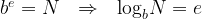${b^e} = N \,\,\,\, \Rightarrow \,\,\,\, {\log _b}N = e$
or in words,
 if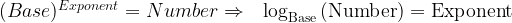${(Base)^{Exponent}} = Number \Rightarrow \,\,\,\, {\log _{{\rm{Base}}}}\left( {{\rm{Number}}} \right) = {\rm{Exponent}}$
Thus, logs are just a way of expressing relations involving powers.
As an exercise, show that${\log _{2\sqrt 2 }}32.\left( {{4^{\dfrac{1}{5}}}} \right) = 3.6$
We’ll now discuss some properties that logs satisfy:
(1)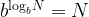${b^{{{\log }_b}N}} = N$
This follows by definition.
(2)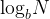${\log _b}N$ is defined only for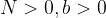$N > 0, b > 0$ and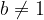$b \ne 1$. We’ll try to understand why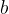$b$ cannot equal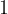$1$:$1$ raised to any power will always equal$1$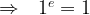$\Rightarrow \,\,\,\, {1^e} = 1$ for all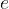$e$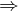$\Rightarrow$ If we try to calculate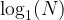${\log _1}(N)$ we see that there can be no value possible for this log, since no matter what power you raise$1$ to, you’ll never obtain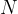$N$. Thus,$1$ is not a valid base for logs.
(3)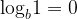${\log _b}1 = 0$ for any base$b$. This is because any number raised to power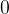$0$ is$1$, so that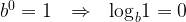${b^0} = 1 \,\,\,\, \Rightarrow \,\,\,\, {\log _b}1 = 0$
(4) Log of a product
One of the most useful properties of logs is this: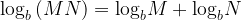${{{\log }_b}\left( {MN} \right) = {{\log }_b}M + {{\log }_b}N}$
that is,. the log of a product is equal to the sum of the individual logs. Let us first see the justification of this property.
Assume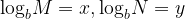${\log _b}M = x,{\log _b}N = y$$\Rightarrow \,\,\,\, {b^x} = M,\,\,{b^y} = N$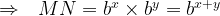$\Rightarrow \,\,\,\, MN = {b^x} \times {b^y} = {b^{x + y}}$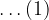$\ldots(1)$$\Rightarrow \,\,\,\, {\log _b}\left( {MN} \right) = x + y = {\log _b}M + {\log _b}N$
Thus, notice carefully that this property is nothing but a manifestation of the fact that when two exponential terms with the same base are multiplied, their powers add, as shown in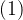$(1)$. This is something you must always keep in mind.
Why is this property so useful? Well, it lets us reduce large multiplications to additions. More on this soon.
(5) Log of a dfraction
Analogous to the product property, we’ve the division property: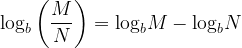${\log _b}\left( {\dfrac{M}{N}} \right) = {\log _b}M - {\log _b}N$
As the alert reader might be expecting, this property is a result of the fact that when two exponential terms with the same base are divided, their powers subtract. Let us prove this property explicitly now.
Assuming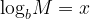${\log _b}M = x$ and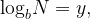${\log _b}N = y,$ we have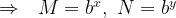$\Rightarrow \,\,\,\, M = {b^x},\,\,N = {b^y}$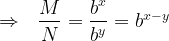$\Rightarrow \,\,\,\, \dfrac{M}{N} = \dfrac{{{b^x}}}{{{b^y}}} = {b^{x - y}}$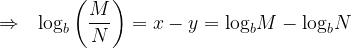$\Rightarrow \,\,\,\, {\log _b}\left( {\dfrac{M}{N}} \right) = x - y = {\log _b}M - {\log _b}N$
As with the multiplication property, this property lets us convert divisions into subtractions and simplifies calculations, as we’ll soon see.
(6) Log of an exponential term
This property states that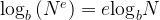${{{\log }_b}\left( {{N^e}} \right) = e{{\log }_b}N}$
that is, the log of${N^e}$ to any base is$e$ times the log of$N$ to the same base. This is again straightforward to justify:
Assume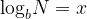${\log _b}N = x$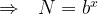$\Rightarrow \,\,\,\, N = {b^x}$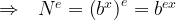$\Rightarrow \,\,\,\, {N^e} = {\left( {{b^x}} \right)^e} = {b^{ex}}$$\Rightarrow \,\,\,\, {\log _b}\left( {{N^e}} \right) = ex = e{\log _b}N$
Thus, this property is a consequence of the fact that when an exponential term is raised to another power, the two powers multiply.
We’ll now understand how the last three properties help us with calculations.